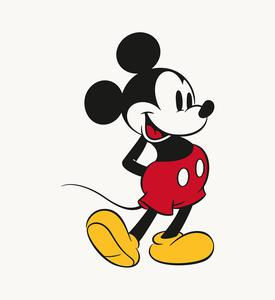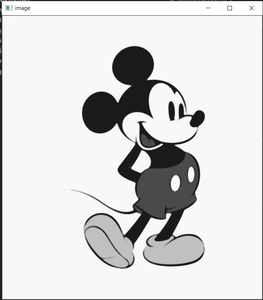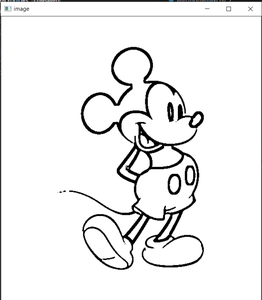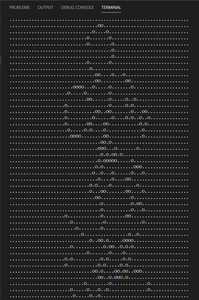Related Articles

# Python script to generate dotted text from any image

• Last Updated : 24 Jan, 2021

Prerequisites: Python OpenCV

In this article, we will see how we can use Python3 to create a dotted text that represents an image. We use such type of texts in messages and social media and generating them is an interesting image processing task.

Attention geek! Strengthen your foundations with the Python Programming Foundation Course and learn the basics.

To begin with, your interview preparations Enhance your Data Structures concepts with the Python DS Course. And to begin with your Machine Learning Journey, join the Machine Learning - Basic Level Course

This task will need us to define the edges of the given image. After that we can use any symbol like ‘.’, ‘o’ or ‘x’ to display the bright and dark pixels.

• First create a new python script and install open CV.
`python3 -m pip install opencv-python --user`
• Now import cv2 and load the image you want to convert. Here the image name is “mic.jpg” and it is in the same directory. We need to use the image in grayscale mode, so give an extra parameter to load in grayscale mode.
```# import the required modules
import cv2

• We can reduce the noise in the image so that the round corners and edges are detected smoothly. We can use medianblur() method of cv2 to achieve that.
```# Apply median blur
img = cv2.medianBlur(img,5)```
• To detect the edges, we have various kind of thresholding functions available in openCV. But the most compatible one in this case is ADAPTIVE_THRESH_MEAN_C. Additionally, we can check other threshold functions too.
```# Apply MEAN thresholding to get refined edges

Now we have an image having only edges. We can iterate over our image to print the bright and dark points and that will generate the dotted text for us. If the image is too large we can use cv2.resize() to shrink the image so that it can be used.

Implementation :

## Python3

 `# Python script to convert the given image``# into a dotted text using opencv`` ` `# import the required modules``import` `cv2`` ` `# Read the image``img ``=` `cv2.imread(``'mic.jpg'``, ``0``)`` ` `# Apply median blur``img ``=` `cv2.medianBlur(img, ``5``)`` ` `# Apply MEAN thresholding to get refined edges``image ``=` `cv2.adaptiveThreshold(``    ``img, ``255``, cv2.ADAPTIVE_THRESH_MEAN_C, cv2.THRESH_BINARY, ``11``, ``2``)`` ` `# Convert the image into a compatible size``# We will use 60 pixels wide image so that text``# fits in the console`` ` `# Preserve the ratio``ratio ``=` `len``(image)``/``len``(image[``0``])``# Assign new width and calculate new height``new_width ``=` `60``new_height ``=` `int``(ratio``*``new_width)``# Resize the image``image ``=` `cv2.resize(image, (new_height, new_width))`` ` `# Iterate over the array and print the dark pixels``# or we can use any other symbol too.``for` `i ``in` `range``(``len``(image)):``    ``for` `j ``in` `range``(``len``(image[``0``])):``        ``print``(``"o"` `if` `image[i, j] < ``100` `else` `"."``, end``=``"")``    ``print``()`
• Original Image :• Gray scale image after applying median blur :• Image after applying Adaptive mean threshold :• Final output printed on console :My Personal Notes arrow_drop_up